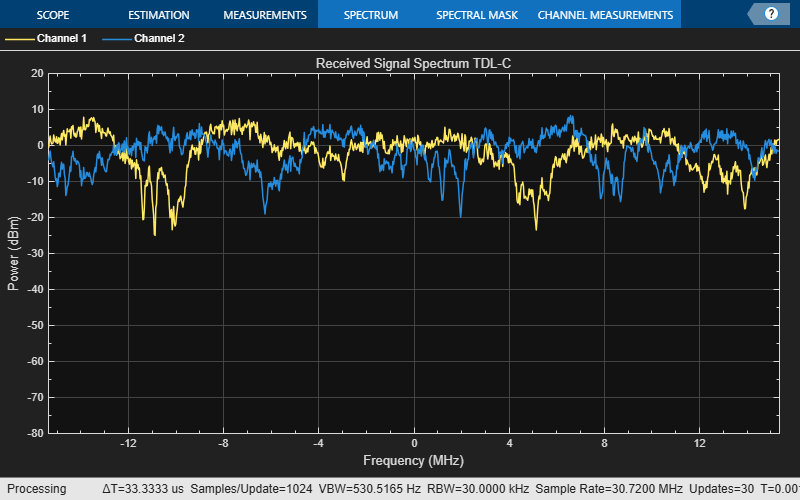# Transmission Over MIMO Channel Model with Delay Profile TDL

Display the waveform spectrum received through a tapped delay line (TDL) multi-input/multi-output (MIMO) channel model from TR 38.901 Section 7.7.2 using an `nrTDLChannel` System object.

Define the channel configuration structure using an `nrTDLChannel` System object. Use delay profile TDL-C from TR 38.901 Section 7.7.2, a delay spread of 300 ns, and UE velocity of 30 km/h:

```v = 30.0; % UE velocity in km/h fc = 4e9; % carrier frequency in Hz c = physconst('lightspeed'); % speed of light in m/s fd = (v*1000/3600)/c*fc; % UE max Doppler frequency in Hz tdl = nrTDLChannel; tdl.DelayProfile = 'TDL-C'; tdl.DelaySpread = 300e-9; tdl.MaximumDopplerShift = fd;```

Create a random waveform of 1 subframe duration with 1 antenna.

```SR = 30.72e6; T = SR * 1e-3; tdl.SampleRate = SR; tdlinfo = info(tdl); Nt = tdlinfo.NumTransmitAntennas; txWaveform = complex(randn(T,Nt),randn(T,Nt));```

Transmit the input waveform through the channel.

`rxWaveform = tdl(txWaveform);`

```analyzer = dsp.SpectrumAnalyzer('SampleRate',tdl.SampleRate,... 'AveragingMethod','Exponential','ForgettingFactor',0.99 ); analyzer.Title = ['Received Signal Spectrum ' tdl.DelayProfile]; analyzer(rxWaveform);```